Equations/Expressions
Exponents
Order of Operations
Combine Like Terms
Exponent Rules
100

Simplify the following (distribute):

8(3+5)

64

100

24

16

100

Simplify the following expression: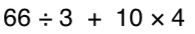100

-4 + -5

-9100

5x + 7x

12x

100

(x2) (x3) = ?

x5

200

solve for the value of p:

p-54 =36

90

200

What is 9^3 (exponent)

93 =  9 x 9 x 9 =  729

200

Simplify the following expression: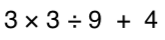200

14 + -12

2

https://www.khanacademy.org/math/arithmetic/arith-review-negative-numbers/arith-review-add-negatives-intro/v/adding-negative-numbers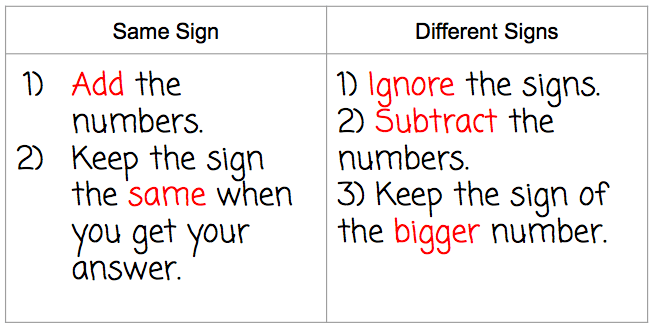200

3x + 6 + 7x + 3

10x + 9

200

(8x3)(2x5) = ?

16x8

300

Solve for the value of x:

3x+1=-8

x=-3

300

what is 2^5

25= 32

300

3 + 42 x (3 + 2)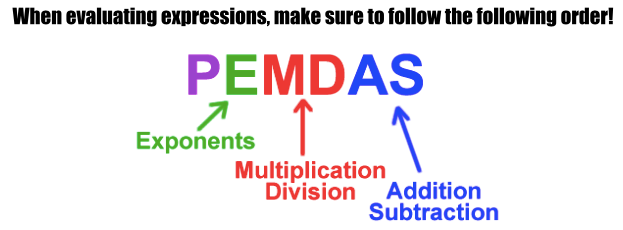=3+16 x 5

=3 + 80

=83

300

-10 + 15

5300

8 + 4x + 1 - 12 + 5x

9x - 3

300

(h2)= ?

h6

400

Distribute the following:

7(x-3)

7x-21

400

What is the value of:

84

4,096

400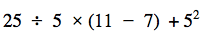=5 x 4 +25

=20+25

=45

400

-18 + (-12) =

-30

400

-11j + 16 - 22j - 27 + 5j

-18j - 11

400

When multiplying monomials with the same base,  we should _______________ the exponents

500

Multiply the following binomials:

(x-3)(x+4)

x+ x - 12

500

What is the value of:

23 + 32 - 42

500=27+9/3

=27+3

= 30

500

6 + (-2 + -5)

-1

500

Combine like terms:

9y + 3 - 4x -2y - 3x - 5

7y - 7x - 2

OR

-7x + 7y - 2

500

(–5xy)(4x2)(y4)

-20x3y5

Click to zoom